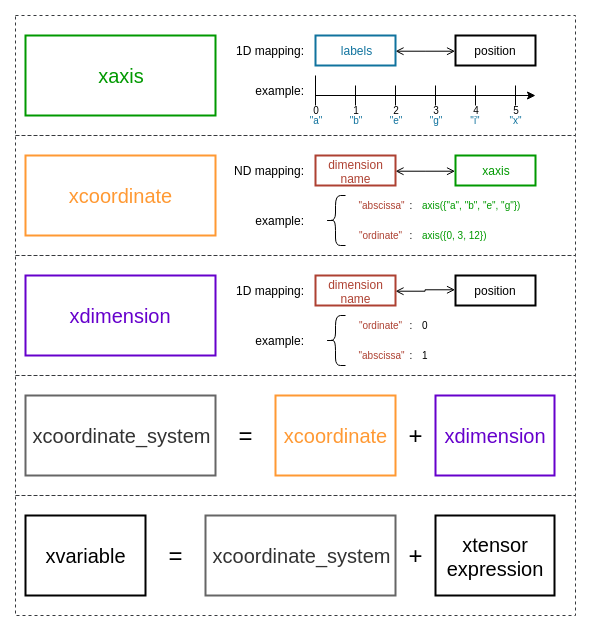# Data structures¶

## Axes¶

An axis is a mapping of labels to positions in a given dimension. It is the equivalent of the `index` object from pandas. xframe supports many types of labels, the most common are strings, char, integers and dates. An axis is created from a list of labels, a builder function is provided so the type of the axis can be inferred. The following example illustrates the two main ways of creating an axis:

```using saxis_type = xf::xaxis<xf::fstring, std::size_t>;

saxis_type s1({ "a", "b", "d", "e" });
auto s2 = xf::axis({ "a", "b", "d", "e" });
// s1 and s2 are similar axes
```

It is also possible to create an axis given the size of the axis or the start, stop and step:

```auto s3 = xf::axis(5);        // == xf::axis({ 0, 1, 2, 3, 4 });
auto s4 = xf::axis(2, 7);     // == xf::axis({ 2, 3, 4, 5, 6 });
auto s5 = xf::axis(0, 10, 2); // == xf::axis({ 0, 2, 4, 6, 8 });
auto s6 = xf::axis("a", "d"); // == xf::axis({ "a", "b", "c" });
```

The axis API is similar to the one of a constant `std::map` that throws an exception when asked a missing key:

```std::size_t i0 = s1["a"];
try
{
std::size_t i1 = s1["c"];
}
catch(std::exception& e)
{
// The exception will be catch since "c" is not a label of s1
std::cout << e.what() << std::endl;
}
```

`xaxis` also provides iterators and methods to compute the union and the intersection of axes. However a user rarely needs to manipulate the axes directly, the most common operation is to create them and then store them in a coordinate system.

## Coordinates¶

Coordinates are mappings of dimension names to axes. xframe provides different methods to easily create them:

```using coordinate_type = xf::xcoordinate<xf::fstring>;

coordinate_type c1({{"group", xf::axis({"a", "b", "d", "e"})},
{"city",  xf::axis({"London", "Paris", "Brussels"})}});
auto c2 = xf::coordinate({{"group", xf::axis({"a", "b", "d", "e"})},
{"city",  xf::axis({"London", "Paris", "Brussels"})}});
// c1 and c2 are similar coordinates
```

Note

The builder function `xf::coordinate` converts the `const char*` arguments to `fstring` and returns a `xcoordinate<fstring>` object. You can modify this behavior by specifying the key type of the coordinate as the first template parameter of the `coordinate` function: `auto c2 = xf::coordinate<std::string>({{"group", xf::axis({"a", "b", "d", "e"})}, ...});`

`xnamed_axis` allows to store a dimension name - axis pair that you can reuse in different coordinates objects; if you want to create a coordinate object from a named axis, all the arguments must be named axes; fortunately, a `xnamed_axis` can be created in place, as shown below:

```// This object will be used in different coordinates objects
auto a1 = xf::named_axis("igroup", xf::axis({1, 2, 4, 5})});

auto c1 = xf::coordinate<xf::fstring>(a1, xf::named_axis("city", xf::axis({"London", "Parid", "Brussels"})));
auto c2 = xf::coordinate<xf::fstring>(a1, xf::named_axis("country", xf::axis({"USA", "Japan"})));
```

As you can notice, coordinates objects can store axes with different label types. By default, these types are `int`, `std::size_t`, `char` and `xf::fstring`, you can specify a different type list:

```using coordinate_type = xf::xcoordinate<xf::fstring, xtl::mpl::vector<int, std::string>>;

coordinate_type c({{"group", xf::axis({"a", "b", "d", "e"})},
{"city",  xf::axis({"London", "Paris", "Brussels"})}});
```

## Dimension¶

A dimension object is the mapping of the dimension names to the dimension positions in the data tensor. Creating a `xdimension` is as simple as creating an `xcoordinate` or an `xaxis`:

```using dimension_type = xf::xdimension<xf::fstring>;

dimension_type dim1({"city", "group"});
auto dim2 = xf::dimension({"city", "group"});
// dim1 and dim2 are similar dimensions
```

`xdimension` provides an API similar to `xaxis` and therefore can be considered as a special axis. Together a dimension object and a coordinate object form a coordinate system which maps labels and dimension names to indexes in the data tensor.

Note

Like `xf::coordinate`, the builder function `xf::dimension` converts the `const char*` arguments to `fstring` and returns a `xdimension<fstring>` object. You can modify this behavior by specifying the label type of the dimension as the first template parameter of the `dimension` function: `auto d = xf::dimension<std::string>({"city", "group"});`

## Variables¶

A variable is a data tensor with a coordinate system, that is an `xcoordinate` object and an `xdimension` object. It is the C++ equivalent of the `xarray.DataArray` Python class. `xvariable` provides many constructors:

```using coordinate_type = xf::xcoordinate<xf::fstring>;
using dimension_type = xf::xdimension<xf::fstring>;
using variable_type = xvariable<double, coordinate_type>;

data_type d = xt::eval(xt::random::rand({3, 4}));
auto c = xf::coordinate({{"group", xf::axis({"a", "b", "d", "e"})},
{"city",  xf::axis({"London", "Paris", "Brussels"})}});
auto dim = xf::dimension({"city", "group"});

variable_type v1(d, c, dim);

// Coordinates and dimension can be built in place
variable_type v2(d, xf::coordinate({{"group", xf::axis({"a", "b", "d", "e"})},
{"city",  xf::axis({"London", "Paris", "Brussels"})}}),
xf::dimension({"city", "group"}));
```

The data parameter can be omitted, in that case the variable creates an uninitialized data tensor:

```variable_type v3(c, dim);

variable_type v4(xf::coordinate({{"group", xf::axis({"a", "b", "d", "e"})},
{"city",  xf::axis({"London", "Paris", "Brussels"})}}),
xf::dimension({"city", "group"}));
```

A variable can also be created from a map of axes and a list of dimension names:

```variable_type::coordinate_map coord_map;
coord_map["group"] = xf::axis({"a", "b", "d", "e"});
coord_map["city"]  = xf::axis({"London", "Paris", "Brussels"});
dimension_type::label_list dim_list = {"group", "city"};

variable_type v5(d, coord_map, dim_list);
variable_type v6(coord_map, dim_list);
```

If the dimension object is omitted, the dimension mapping is inferred from the coordinate object. In the code below, the mapping is different from the previous defined variables, `group` is the name of the first dimension and `city` is the name of the second one:

```variable_type v7(d, {{"group", xf::axis({"a", "b", "d", "e"})},
{"city",  xf::axis({"london", "Paris", "Brussels"})}});

// variable with same coordinate system but uninitialized data
variable_type v8({{"group", xf::axis({"a", "b", "d", "e"})},
{"city",  xf::axis({"london", "Paris", "Brussels"})}});
```

xframe also provides builder functions, so that the type of the variable can be inferred:

```auto v10 = variable(d, c, dim);
auto v11 = variable(d, xf::coordinate({{"group", xf::axis({"a", "b", "d", "e"})},
{"city",  xf::axis({"London", "Paris", "Brussels"})}}),
xf::dimension({"city", "group"}));

auto v12 = variable(c, dim);
auto v13 = variable(xf::coordinate({{"group", xf::axis({"a", "b", "d", "e"})},
{"city",  xf::axis({"London", "Paris", "Brussels"})}}),
xf::dimension({"city", "group"}));
```

## Summary¶